﻿ Inequalities of Type Hermite-Hadamard for Fractional Integrals via Differentiable Convex Functions

### Inequalities of Type Hermite-Hadamard for Fractional Integrals via Differentiable Convex Functions

P. O. Mohammed

Turkish Journal of Analysis and Number Theory

## Inequalities of Type Hermite-Hadamard for Fractional Integrals via Differentiable Convex Functions

P. O. MohammedDepartment of Mathematics, College of Education, Sulaimani University, Sulaimani, Kurdistan Region, Iraq

### Abstract

In this paper, we established some new Hermite-Hadamard-type inequalities for differentiable convex functions via Reimann-Liouville fractional integrals. Moreover, our results improve and extend the corresponding ones in the literature.

• P. O. Mohammed. Inequalities of Type Hermite-Hadamard for Fractional Integrals via Differentiable Convex Functions. Turkish Journal of Analysis and Number Theory. Vol. 4, No. 5, 2016, pp 135-139. http://pubs.sciepub.com/tjant/4/5/3
• Mohammed, P. O.. "Inequalities of Type Hermite-Hadamard for Fractional Integrals via Differentiable Convex Functions." Turkish Journal of Analysis and Number Theory 4.5 (2016): 135-139.
• Mohammed, P. O. (2016). Inequalities of Type Hermite-Hadamard for Fractional Integrals via Differentiable Convex Functions. Turkish Journal of Analysis and Number Theory, 4(5), 135-139.
• Mohammed, P. O.. "Inequalities of Type Hermite-Hadamard for Fractional Integrals via Differentiable Convex Functions." Turkish Journal of Analysis and Number Theory 4, no. 5 (2016): 135-139.

 Import into BibTeX Import into EndNote Import into RefMan Import into RefWorks

### 1. Introduction

The following definition is well known in the literature.

Definition 1.1. [7, 8] A function: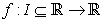is said to beconvex on the interval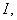if for all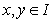and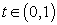satisfies the following inequality: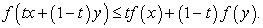Many significant inequalities have been studied for the class of convex functions, but the most important is the following inequality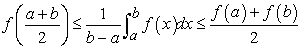(1)

that is known as Hermite-Hadamard inequality . For more systematic information, please refer to the monographs [3-8] and closely related references therein.

In what follows we recall the following definition .

Definition 1.2. Let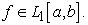The left Riemann-Liouville fractional integrals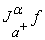and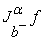of order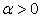are defined by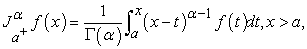and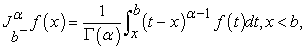respectively. Here,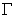is the gamma function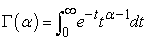and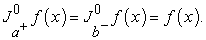Tomar et. al.  established the following Hermite-Hadamard type inequalities via Riemann-Liouville fractional integrals:

Lemma 1.1. Letbe twice differentiable mapping on the interior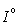of an interval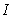such that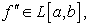where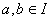with a < b. Then for each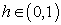andthe following equality holds: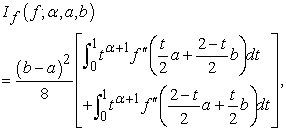(2)

where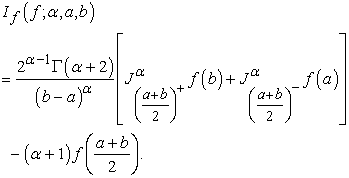Theorem 1.1. The assupmtions of Lemma 1.1 are satisfied. If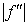is convex on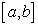, then for eachthe following inequality holds: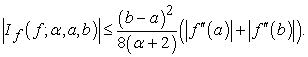(3)

Theorem 1.2. The assupmtions of Lemma 1.1 are satisfied. If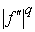is convex onfor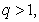then the following inequality holds: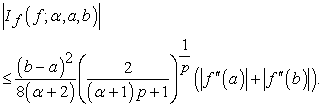(4)

where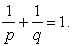Theorem 1.3. Let the assupmtions of Lemma 1.1 be satisfied. Ifis convex onforthen the following inequality holds: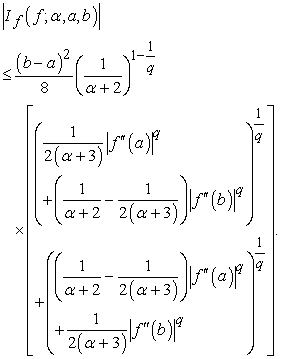(5)

### 2. Main Results

Lemma 2.1. Letbe twice differentiable mapping on the interiorof an intervalsuch thatwherewith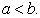Then for eachandthe following equality holds: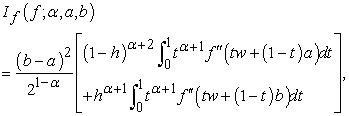(6)

where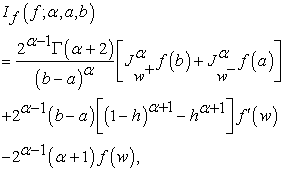and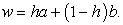Proof. Denoting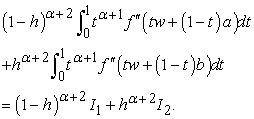(7)

Integrating by parts twice and changing variable of definite integral, we have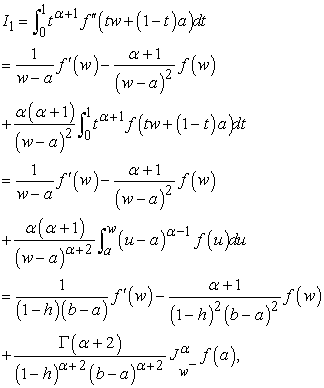(8)

and similarly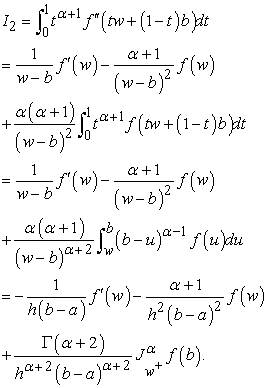(9)

Using equations (8)-(9) in (7) and by the simple calculations, we obtain the desired result.

Remark 2.1. If we take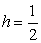in Lemma 2.1, then the identity (6) reduces to the identity (2) which was proved by Tomar et. al. .

Theorem 2.1. The assupmtions of Lemma 1.1 are satisfied. Ifis convex on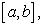then for eachthe following inequality holds: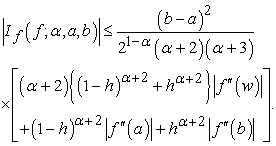(10)

Proof. From Lemma 2.1, we have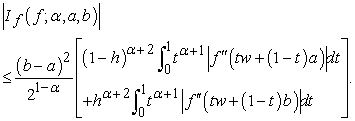Now, sinceis convex, we have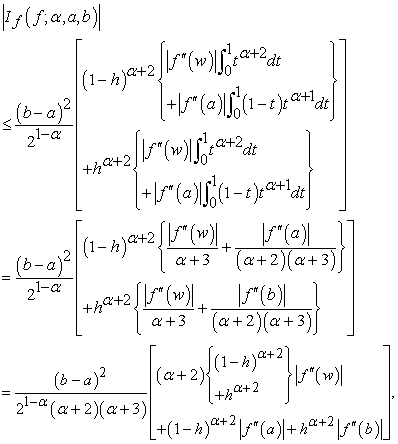and this ends the proof.

Remark 2.2. If we takein Theorem 2.1, then inequality (10) reduces to (3).

Theorem 2.2. The assupmtions of Lemma 1.1 are satisfied. Ifis convex onforthen the following inequality holds: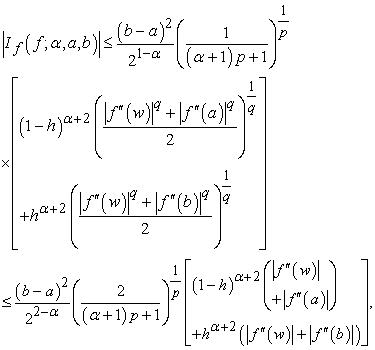(11)

whereProof. From Lemma 2.1 and using the well known Hölder integral inequality, we have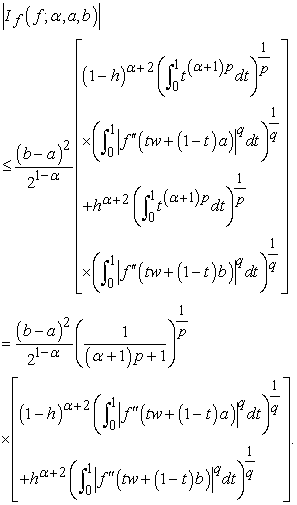(12)

Sinceis convex, then we have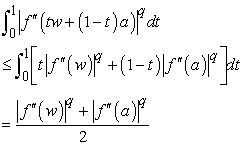(13)

and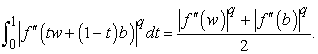(14)

Thus, if we use (13) and (14) in (12), we obtain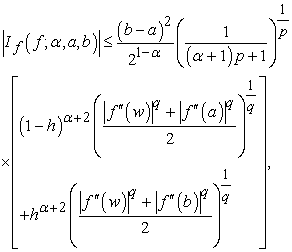this completes the proof of first part of the Theorem.

Let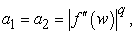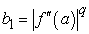and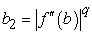for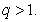Using the fact that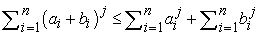for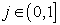and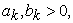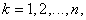we find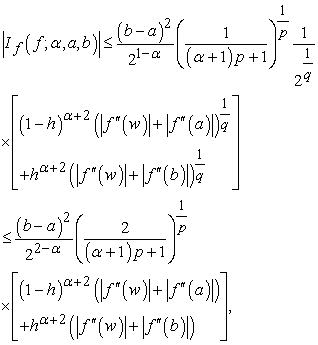which completes the proof of last part of the Theorem. This completes the proof.

Remark 2.3. If we choosein Theorem 2.2, then inequality (11) reduces to (4).

Theorem 2.3. Let the assumptions of Lemma 1.1 be satisfied. Ifis convex onforthen the following inequality holds: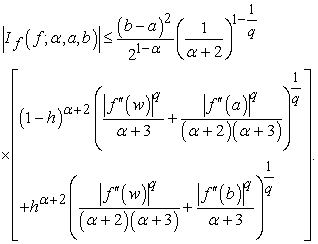(15)

Proof. From Lemma 2.1 and using the well known power mean inequality, we have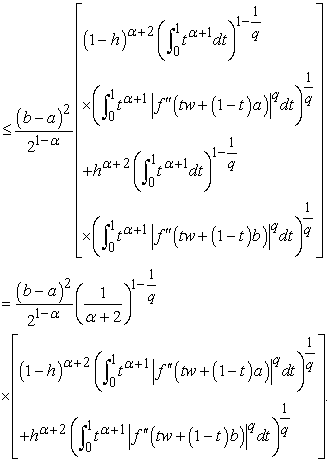(16)

Sinceis convex, then we have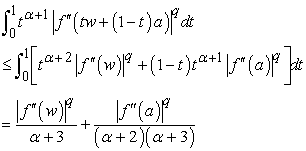(17)

and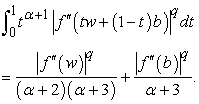(18)

Combining equations (16), (17) and (18), we obtain inequality (15) as required.

Remark 2.4. If we choosein Theorem 2.3, then inequality (15) reduces to (5).

### References

  J. Hadamard, Étude sur les propriétés des fonctions entières en particulier d'une function considérée par Riemann, J Math Pures Appl, 58 (1893), 171-215.In article  M. Tomar, E. Set and M. Z. Sarıkaya, Hermite-Hadamard type Riemann-Liouville fractional integral inequalities for convex functions, AIP Conference Proceedings 1726, 020035 (2016).In article View Article  Z. Dahmani, On Minkowski and Hermite-Hadamad integral inequalities via fractional integration, Annals of Functional Analysis, 1(1) (2010), 51-58.In article View Article  M. Tunç, Y. Subas and I. Karabayir, On some Hadamard type inequalities for MTconvex functions, Int. J. Open Probl. Comput. Sci. Math., 6 (2013), 102-113.In article View Article  S. Qaisar and S. Hussain, Generalization of integral inequalities of the type of Hermite-Hadamard through invexity, Malaya J. Mat., 3(1) (2015), 99-109.In article  J. Park, Fractional Hermite-Hadamard-like Type Inequalities for Convex Functions, International Journal of Mathematical Analysis, 9(29) (2015), 1415-1429.In article View Article  Z. Lin, J. Wang and W. Wei, Fractional Hermite-Hadamard inequalities through r-convex functions via power means, Ser. Math. Inform., 30(2) (2015), 129-145.In article  M. Z. Sarikaya, H. Yaldiz and H. Budak, Some integral inequalities for convex stochastic processes, Acta Math. Univ. Comenianae, LXXXV(1) (2016), 155-164.In article  I. Podlubni, Fractional Differential Equations, Academic Press, San Diego, 1999.In article